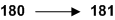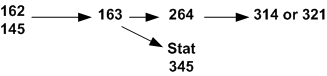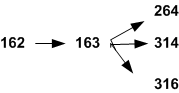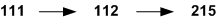# Mathematics and Statistics

Terry A. Loring, Chairman
Department of Mathematics and Statistics
Science and Math Learning Center
MSC01 1115
1 University of New Mexico
Albuquerque, NM 87131-0001
(505) 277-4613

Professors
Alexandru Buium, Ph.D., University of Bucharest (Romania)
Ronald Christensen, Ph.D., University of Minnesota
Pedro F. Embid, Ph.D., University of California (Berkeley)
Gabriel Huerta, Ph.D., Duke University
Jens Lorenz, Ph.D., University of Münster (Germany)
Terry A. Loring, Ph.D., University of California (Berkeley)
Pavel M. Lushnikov, Ph.D., L.D. Landau Institute for Theoretical Physics of the Russian Academy of Sciences
Michael J. Nakamaye, Ph.D., Yale University
Monika Nitsche, Ph.D., University of Michigan
Maria C. Pereyra, Ph.D., Yale University
Deborah L. Sulsky, Ph.D., New York University

Associate Professors
Matthew Blair, Ph.D., University of Washington
Alexander O. Korotkevich, Ph.D., L.D. Landau Institute for Theoretical Physics of the Russian Academy of Sciences
Stephen Lau, Ph.D., University of North Carolina (Chapel Hill)
Yan Lu, Ph.D., Arizona State University
Kristin Umland, Ph.D., University of Illinois (Chicago)
Dimiter Vassilev, Ph.D., Purdue University
Janet Vassilev, Ph.D., University of California, Los Angeles
Helen Wearing, Ph.D., Heriot-Watt University (Edinburgh, Scotland)
Guoyi Zhang, Ph.D., University of Arizona
Maxim Zinchenko, Ph.D., University of Missouri

Assistant Professors
Daniel Appelo, Ph.D., Royal Institute of Technology (Sweden)
Jehanzeb H. Chaudhry, Ph.D., University of Illinois Urbana - Champaign
James Degnan, Ph.D., University of New Mexico
Erik B. Erhardt, Ph.D., University of New Mexico
Hongnian Huang, Ph.D., University of Wisconsin, Madison
Li Li, Ph.D., University of South Carolina
Mohammad Motamed, Ph.D., Royal Institute of Technology (Sweden)
Matthew Pennybacker, Ph.D., University of Arizona, Tucson
Anna Skripka, Ph.D., University of Missouri

Lecturers
Timothy Berkopec, M.S., University of Illinois, Urbana
Jurg Bolli, M.S., University of Zurich (Switzerland)
Karen Champine, M.A., University of New Mexico
Nina Greenberg, M.S., University of New Mexico
Derek Martinez, Ph.D., University of New Mexico
Karen Sorensen-Unruh, M.S., University of New Mexico

Professors Emeriti
Richard C. Allen, Ph.D., University of New Mexico
Charles P. Boyer, Ph.D., Pennsylvania State University
Evangelos A. Coutsias, Ph.D., California Institute of Technology
James A. Ellison, Ph.D., California Institute of Technology
Roger C. Entringer, Ph.D., University of New Mexico
Archie G. Gibson, Ph.D., University of Colorado
Frank L. Gilfeather, Ph.D., University of California (Irvine)
Nancy A. Gonzales, Ed.D., Harvard University
Richard J. Griego, Ph.D., University of Illinois
Liang-Shin Hahn, Ph.D., Stanford University
Reuben Hersh, Ph.D., New York University
Wojciech Kucharz, Ph.D., Jagiellonian University (Krakow, Poland)
James V. Lewis, Ph.D., University of California (Berkeley)
Richard C. Metzler, Ph.D., Wayne State University
Cornelis W. Onneweer, Ph.D., Wayne State University
Pramod K. Pathak, Ph.D., Indian Statistical Institute
Clifford R. Qualls, Ph.D., University of California (Riverside)
Ronald M. Schrader, Ph.D., Pennsylvania State University
Stanly L. Steinberg, Ph.D., Stanford University
Alexander P. Stone, Ph.D., University of Illinois
William J. Zimmer, Ph.D., Purdue University

#### Introduction

Mathematics is fundamental to the formulation and analysis of scientific theories, is a rich and independent field of inquiry, and its study is excellent preparation for life in our highly specialized society. Active research throughout the mathematical subdisciplines, spurred on in part by advances in computing technology, leads to new perspectives and applications. The major in mathematics combines broad study of fundamental theories with in-depth investigation of particular subjects chosen from pure, applied and computational mathematics. A degree in mathematics, either alone or in combination with study in another field, is excellent preparation for careers in industry, universities and research institutes.

Statistics is the science of collecting and analyzing data. Statisticians interact with researchers in all the various disciplines of science, engineering, medicine, social science and business to develop scientifically sound methods in those areas. Most course work in the department is devoted to understanding current methods and the reasoning behind them. A degree in statistics prepares students for careers in industry, government, universities and research institutes, as well as being excellent preparation for professional programs in medicine, law, business administration and public policy and administration.

High School Students: In order to graduate from the University of New Mexico, all students are required to take a minimum of 3 credits of mathematics course work at the college algebra (MATH 121) level or above. To prepare for this level of study, high school students must take two years of algebra and one year of geometry prior to admission. Students should take mathematics during their senior year of high school, and also take the ACT or SAT examination during that year, for the best preparation and placement into mathematics courses at the University of New Mexico. Students planning to major in any scientific or technological field should take more advanced mathematics courses in high school. Placement in Mathematics or Statistics courses at UNM is based on the most recent ACT/SAT Math scores.

A beginning student who wishes to take MATH 163 or a more advanced course must have College Board Advanced Placement scores as described in the Admissions section of this Catalog.

A student who wishes to enroll in a course requiring a prerequisite must earn a grade of "C" (not "C-") or better in the prerequisite course.

#### Flow Chart

A student’s preparation determines the starting course in any sequence.

Calculus for biological and social sciencesMathematics major sequenceStatistics major sequenceEngineering sequenceElementary education sequenceRestrictions

1. Credit not allowed for both MATH 162 and 180.
2. Credit not allowed for both MATH 163 and 181.
3. Credit not allowed for both MATH 314 and 321.
4. Credit not allowed for both MATH 401 and 501.
5. Credit not allowed for both MATH 322 and 422.
6. Students who have credit for any courses numbered MATH 121 and above may not take MATH 101 -103 for credit.
7. Students who have credit for any courses numbered 162 and above may not take MATH 101-103, 121, 123 or 150 for credit. (Students with MATH 180/181 may take MATH 123 for credit).
8. Students who have credit for any courses numbered MATH 121 or above may also take MATH 129 and/or STAT 145.
9. A student may not take an examination to validate credit in mathematics courses except those offered through UNM Testing Center.
10. Mathematics or Statistics course work dating back more than five years cannot automatically be counted as fulfillment of a prerequisite. Students with older course work who feel they have retained subject knowledge are encouraged to take the placement exam offered through the University of New Mexico Testing Center.

Undergraduate courses in Mathematics (MATH) may be categorized as Introductory Course, or as Courses for Teachers and Education Students. Courses in these categories are identified in parenthesis at the end of the course description according to the following legend:

Introductory Courses (I), Courses for Teachers and Education Students (T).

### Courses

MATH 101. Intermediate Algebra Part 1. (1)

MATH 102. Intermediate Algebra Part 2. (1)

MATH 103. Intermediate Algebra Part 3. (1)

MATH 107. Problems in College Algebra. (1)

MATH 110. Problems in Elements of Calculus. (1)

MATH 111. Mathematics for Elementary and Middle School Teachers I. (3)

MATH 112. Mathematics for Elementary and Middle School Teachers II. (3)

MATH 116. Topics in Pre-Calculus Mathematics. (1-6 to a maximum of 12 Δ)

MATH 121. College Algebra. (3)

MATH 123. Trigonometry. (3)

MATH 129. A Survey of Mathematics. (3)

MATH 150. Pre-Calculus Mathematics. (3)

MATH 153. Precalculus and Trigonometry. (5)

MATH 162. Calculus I. (4)

MATH 163. Calculus II. (4)

MATH 180. Elements of Calculus I. (3)

MATH 181. Elements of Calculus II. (3)

MATH 215. Mathematics for Elementary and Middle School Teachers III. (3)

MATH 264. Calculus III. (4)

MATH 305 / 507. Mathematics from a Historical Perspective. (3)

MATH 306 [306 / 506]. College Geometry. (3)

MATH 311. Vector Analysis. (3)

MATH **312. Partial Differential Equations for Engineering. (3)

MATH **313. Complex Variables. (3)

MATH **314. Linear Algebra with Applications. (3)

MATH **316. Applied Ordinary Differential Equations. (3)

MATH **317. Elementary Combinatorics. (3)

MATH **319. Theory of Numbers. (3)

MATH **321. Linear Algebra. (3)

MATH 322. Modern Algebra I. (3)

MATH **327. Introduction to Mathematical Thinking and Discrete Structures. (3)

MATH 338 / 542. Mathematics for Secondary Teachers. (3)

MATH 339 / 543. Topics in Mathematics for Elementary and Middle School Teachers. (1-3, no limit Δ)

MATH 350 / 550. Topics in Mathematics for Secondary Teachers. (1-3, no limit Δ)

MATH **356. Symbolic Logic. (4)

MATH **375. Introduction to Numerical Computing. (3)

MATH 391. Advanced Undergraduate Honors Seminar. (1-3 to a maximum of 8 Δ)

MATH 393. Topics in Mathematics. (3, no limit Δ)

MATH 401 / 501. Advanced Calculus I. (4)

MATH 402 / 502. Advanced Calculus II. (3)

MATH **412. Nonlinear Dynamics and Chaos. (3)

MATH **415. History and Philosophy of Mathematics. (3)

MATH *421. Modern Algebra II. (3)

MATH **422. Modern Algebra for Engineers. (3)

MATH *431 / 535. Introduction to Topology. (3)

MATH **439. Topics in Mathematics. (1-3, no limit Δ)

MATH 441. Probability. (3)

MATH 462 / 512. Introduction to Ordinary Differential Equations. (3)

MATH 463 / 513. Introduction to Partial Differential Equations. (3)

MATH 464 / 514. Applied Matrix Theory. (3)

MATH *466. Mathematical Methods in Science and Engineering. (3)

MATH *471. Introduction to Scientific Computing. (3)

MATH 472 / 572. Fourier Analysis and Wavelets. (3)

MATH 499. Individual Study. (1-3 to a maximum of 6 Δ)

MATH 501 / 401. Advanced Calculus I. (4)

MATH 502 / 402. Advanced Calculus II. (3)

MATH 504. Introductory Numerical Analysis: Numerical Linear Algebra. (3)

MATH 505. Introductory Numerical Analysis: Approximation and Differential Equations. (3)

MATH 507 / 305. Mathematics from a Historical Perspective. (3)

MATH 510. Introduction to Analysis I. (3)

MATH 511. Introduction to Analysis II. (3)

MATH 512 / 462. Introduction to Ordinary Differential Equations. (3)

MATH 513 / 463. Introduction to Partial Differential Equations. (3)

MATH 514 / 464. Applied Matrix Theory. (3)

MATH 519. Selected Topics in Algebra and Number Theory. (3, no limit Δ)

MATH 520. Abstract Algebra I. (3)

MATH 521. Abstract Algebra II. (3)

MATH 530. Algebraic Geometry I. (3)

MATH 531. Algebraic Geometry II. (3)

MATH 532. Algebraic Topology I. (3)

MATH 533. Algebraic Topology II. (3)

MATH 535 / 431. Foundations of Topology. (3)

MATH 536. Introduction to Differentiable Manifolds. (3)

MATH 537. Riemannian Geometry I. (3)

MATH 538. Riemannian Geometry II. (3)

MATH 539. Selected Topics in Geometry and Topology. (3, no limit Δ)

MATH 540. Stochastic Processes with Applications. (3)

MATH 542 / 338. Mathematics for Secondary Teachers. (3)

MATH 543 / 339. Topics in Mathematics for Elementary and Middle School Teachers. (1-3, no limit Δ)

MATH 549. Selected Topics in Probability Theory. (3, no limit Δ)

MATH 550 / 350. Topics in Mathematics for Secondary Teachers. (1-3, no limit Δ)

MATH 551. Problems. (1-3, no limit Δ)

MATH 557. Selected Topics in Numerical Analysis. (3, no limit Δ)

MATH 561. Functions of a Complex Variable I. (3)

MATH 562. Functions of a Complex Variable II. (3)

MATH 563. Measure Theory. (3)

MATH 565. Harmonic Analysis. (3)

MATH 569. Selected Topics in Analysis. (3, no limit Δ)

MATH 570. Singular Perturbations. (3)

MATH 572 / 472. Fourier Analysis and Wavelets. (3)

MATH 576. Numerical Linear Algebra. (3)

MATH 578. Numerical Partial Differential Equations. (3)

MATH 579. Selected Topics in Applied Mathematics. (3, no limit Δ)

MATH 581. Functional Analysis I. (3)

MATH 583. Methods of Applied Mathematics I. (3)

MATH 584. Methods of Applied Mathematics II. (3)

MATH 598. Practicum. (1-6 to a maximum of 6 Δ)

MATH 599. Master's Thesis. (1-6, no limit Δ)

MATH 605. Graduate Colloquium. (1 to a maximum of 4 Δ)

MATH 639. Seminar in Geometry and Topology. (1-3, no limit Δ)

MATH 649. Seminar in Probability and Statistics. (1-3, no limit Δ)

MATH 650. Reading and Research. (1-6 to a maximum of 12 Δ)

MATH 669. Seminar in Analysis. (1-3, no limit Δ)

MATH 679. Seminar in Applied Mathematics. (1-3, no limit Δ)

MATH 689. Seminar in Functional Analysis. (1-3)

MATH 699. Dissertation. (3-12, no limit Δ)

STAT 145. Introduction to Statistics. (3)

STAT 279. Topics in Introductory Statistics. (1 to 3 to a maximum of 3 Δ)

STAT **345. Elements of Mathematical Statistics and Probability Theory. (3)

STAT 427 / 527. Advanced Data Analysis I. (3)

STAT 428 / 528. Advanced Data Analysis II. (3)

STAT 434 / 534. Contingency Tables and Dependence Structures. (3)

STAT 440 / 540. Regression Analysis. (3)

STAT 445 / 545. Analysis of Variance and Experimental Design. (3)

STAT 453 / 553. Statistical Inference with Applications. (3)

STAT 461 / 561. Probability. (3)

STAT 470 / 570. Industrial Statistics. (3)

STAT 472 / 572. Sampling Theory and Practice. (3)

STAT 474 / 574. Biostatistical Methods: Survival Analysis and Logistic Regression. (3)

STAT 476 / 576. Multivariate Analysis. (3)

STAT 477 / 577. Introduction to Bayesian Modeling. (3)

STAT 479. Topics in Statistics. (3, no limit Δ)

STAT 481 / 581. Introduction to Time Series Analysis. (3)

STAT 495. Individual Study. (1-3 to a maximum of 6 Δ)

STAT 520. Topics in Interdisciplinary Biological and Biomedical Sciences. (3, no limit Δ)

STAT 527 / 427. Advanced Data Analysis I. (3)

STAT 528 / 428. Advanced Data Analysis II. (3)

STAT 534 / 434. Contingency Tables and Dependence Structures. (3)

STAT 540 / 440. Regression Analysis. (3)

STAT 545 / 445. Analysis of Variance and Experimental Design. (3)

STAT 546. Theory of Linear Models. (3)

STAT 547. Multivariate Analysis and Advanced Linear Models. (3)

STAT 553 / 453. Statistical Inference with Applications. (3)

STAT 556. Advanced Statistical Inference I. (3)

STAT 557. Advanced Statistical Inference II. (3)

STAT 561 / 461. Probability. (3)

STAT 565. Stochastic Processes with Applications. (3)

STAT 569. Selected Topics in Probability Theory. (3, no limit Δ)

STAT 570 / 470. Industrial Statistics. (3)

STAT 572 / 472. Sampling Theory and Practice. (3)

STAT 574 / 474. Biostatistical Methods: Survival Analysis and Logistic Regression. (3)

STAT 576 / 476. Multivariate Analysis. (3)

STAT 577 / 477. Introduction to Bayesian Modeling. (3)

STAT 579. Selected Topics in Statistics. (3, no limit Δ)

STAT 581 / 481. Introduction to Time Series Analysis. (3)

STAT 586. Nonparametric Curve Estimation and Image Reconstruction. (3)

STAT 590. Statistical Computing. (3)

STAT 595. Problems. (1-3, no limit Δ)

STAT 599. Master's Thesis. (1-6, no limit Δ)

STAT 605. Graduate Colloquium. (1 to a maximum of 4 Δ)

STAT 649. Seminar in Probability and Statistics. (1-3, no limit Δ)

STAT 650. Reading and Research. (1-6 to a maximum of 12 Δ)

STAT 699. Dissertation. (3-12, no limit Δ)

### Keyword Search:

Office of the Registrar

MSC 11 6325
1 University of New Mexico
Albuquerque, NM 87131

Phone: (505) 277-8900
Fax: (505) 277-6809